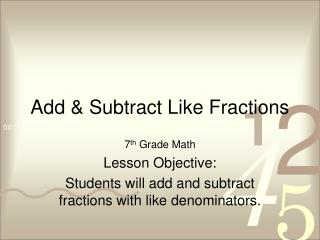DownloadDownload Presentation# Add & Subtract Like Fractions

Télécharger la présentation## Add & Subtract Like Fractions

- - - - - - - - - - - - - - - - - - - - - - - - - - - E N D - - - - - - - - - - - - - - - - - - - - - - - - - - -
##### Presentation Transcript

1. Add & Subtract Like Fractions 7th Grade Math Lesson Objective: Students will add and subtract fractions with like denominators.

2. Vocabulary • Like Fractions • Fractions with common denominators

5. Whiteboard Practice • Add. Write in simplest form.

6. Subtracting Like Fractions • Subtract. Write in simplest form.

7. Subtracting Like Fractions • Subtract. Write in simplest form.

8. Whiteboard Practice • Subtract. Write in simplest form.

9. Real World Example • About 6/100 of the population of the United States lives in Florida. Another 4/100 loves in Ohio. How much more of the U.S. population lives in Florida than in Ohio?

10. Whiteboard Practice • In Mr. Navarro’s first period class, 17/28 of the students got an A on their math test. In his second period class, 11/28 of the students got an A. What fraction more of the students got an A in Mr. Navarro’s first period class than his second period class?

11. Whiteboard Practice • Of the 50 states in the United States, 14 have an Atlantic Ocean coastline and 5 have a Pacific Ocean coastline. What fraction of U.S. states have either an Atlantic Ocean or Pacfic Ocean coastline?